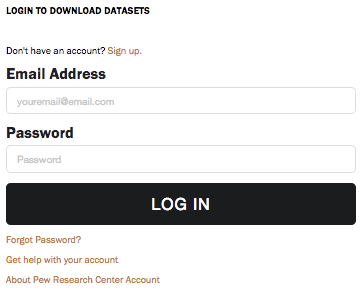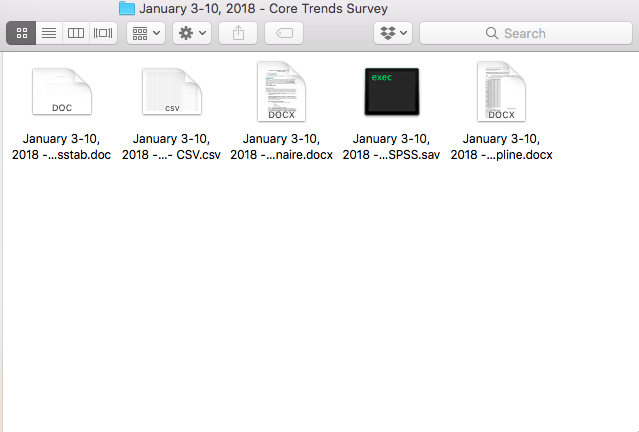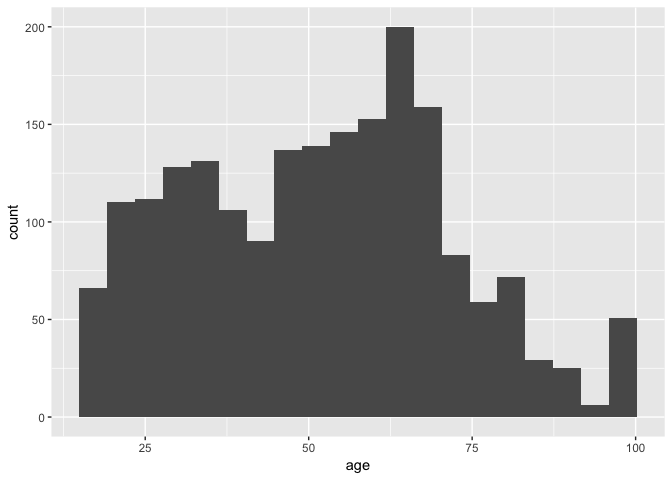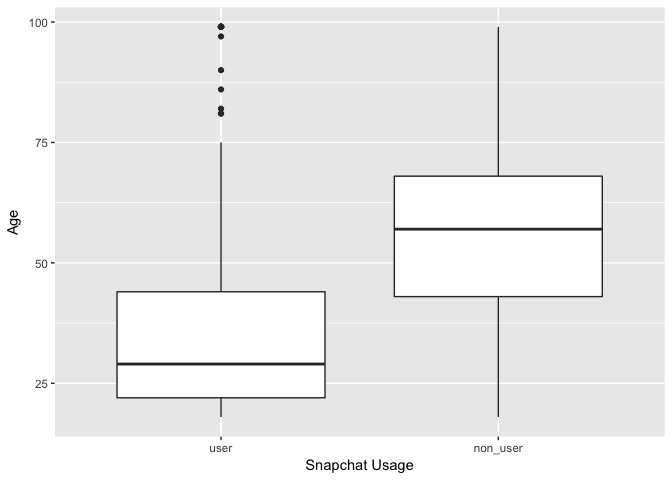Tutorials
tidyverse
+1

Tidy Analysis of Pew Research Data Using R

Learn how to access data from the Pew Research Center, load it into R & then how to explore the data using the Tidyverse ecosystem.

Introduction

The Pew Research Center is a nonpartisan fact tank headquartered in Washington, D.C. Since the center's inception in 2004, it has conducted high quality research in several areas. The P.R.C. currently focuses on nine areas:

• U.S. Politics & Policy
• Journalism & Media
• Internet & Technology
• Science & Society
• Religion & Public Life
• Hispanic Trends
• Global Attitudes & Trends
• Social & Demographic Trends
• Research Methodology

That is a wide range of topics! The P.R.C releases high quality datasets pertaining to all nine areas of research. Data scientists rejoice!

In this tutorial, we will cover how to:

2. Load the data into R
3. Perform exploratory data analysis using tidy tools
4. Conduct a tidy hypothesis test using the infer package

Let's get started!

1. Setting up a Pew Account

In order to download raw datasets from Pew, it is necessary to sign up for a Pew account. Thankfully, this is fairly straightforward to do. To do so, navigate to http://www.pewinternet.org/datasets. You will see the following page for new users:Go ahead and click the link to sign up for an account, and provide the necessary information to register for a Pew account. With account registration out of the way, let's download a dataset and read it into R!

In this tutorial, we're going to explore the Jan. 3-10, 2018 -Core Trends Survey from the Internet & Tech section. When you download the dataset, you will get the following folder:This folder contains the survey data in several formats. For the purpose of this tutorial, we are interested in the .csv file, and the word document containing information about the survey questionnaire. Let's read the data into R:

#First, load the following packages (if you don't have them, use the install.packages() function)
library(tidyverse)
library(infer)
setwd("~/<Your File Path Here>/January 3-10, 2018 - Core Trends Survey")
jan_core_trends_survey <- read_csv("January 3-10, 2018 - Core Trends Survey - CSV.csv")

3. Perform exploratory data analysis using tidy tools

Now that we have read the data into R, let's examine it a bit:

nrow(jan_core_trends_survey)
length(jan_core_trends_survey)
##  2002
length(jan_core_trends_survey)
##  70

The dataset consists of 2002 observations, each with 70 variables. That is a large number of variables. It may be time to consult the questionnaire in order to better understand what types of data were recorded in this survey. This was a telephone opinion survey, where respondents were asked a series of questions about their technology usage, and views about technology. Additional questions to obtain data such as age, and educational attainment were asked as well. In the questionnaire, names for the columns corresponding to questions are provided. For example, answers to the question "Do you use the internet or email, at least occasionally?" are stored in the eminuse column. Let's see what this looks like:

#what values are stored in the eminuse column?
unique(jan_core_trends_survey$eminuse) ##  1 2 8 #first 10 values of age column head(jan_core_trends_survey$age)
##  33 76 99 60 55 58

Well, the age looks like we would expect it to, but what do the values in the eminuse column represent?

Looking at the questionnaire, we see that there is a key for values corresponding which answers they represent:

• 1 = Yes
• 2 = No
• 8 = (VOL.) Don't know
• 9 = (VOL.) Refused

The values in the eminuse column make a lot more sense now! Now that we are getting a better feel for the structure of the data, let's take a look at the distribution of ages in the dataset:

ggplot(jan_core_trends_survey, aes(age)) +
geom_histogram(bins = 20)It looks like the distribution of ages in the dataset is skewed to the left. Does that makes sense when considering what the distribution of ages in the overall population is? Yes! At a given time, a higher proportion of the population is younger, which is in line with our histogram above.

Moving on, there is an interesting series of columns labeled web1a-web1h (ex. web1a, web1b, ...) that represents respondents answers to the following question: "Please tell me if you ever use any of the following social media sites online or on your cell phone." Where:

• web1b = Instagram
• web1d = Snapchat

Let's explore this a little bit. Here is a function to calculate the average ages of users and non-users of these different social media platforms:

avg_user_ages <- function(df, group, var) {
#this step is necessary for tidy evaluation
group <- enquo(group)
var <- enquo(var)
df %>%
select(!!group, !!var) %>%
## we are only looking for 1 (user), or 2 (non-user)
filter(!!group == 1 | !!group == 2) %>%
group_by(!!group) %>%
summarize(avg_age = mean(!!var))
}

You may be unfamiliar with some of the syntax in this function. This is because dplyr functions use tidy evaluation. Excellent documentation for reading more about how tidy evaluation works can be found here.

Using this function, we see:

## # A tibble: 2 x 2
##   web1a avg_age
##   <int>   <dbl>
## 1     1    43.3
## 2     2    53.7
instagram_age <- avg_user_ages(jan_core_trends_survey, web1b, age)

instagram_age
## # A tibble: 2 x 2
##   web1b avg_age
##   <int>   <dbl>
## 1     1    40.9
## 2     2    56.2

## # A tibble: 2 x 2
##   web1c avg_age
##   <int>   <dbl>
## 1     1    48.0
## 2     2    58.3
snapchat_age <- avg_user_ages(jan_core_trends_survey, web1d, age)

snapchat_age
## # A tibble: 2 x 2
##   web1d avg_age
##   <int>   <dbl>
## 1     1    35.7
## 2     2    55.9

Interesting! Perhaps as expected, the average ages for users of all four platforms were substantially lower than their non-user counterparts. For Snapchat, the averages differ by 20 years!!

We can use ggplot to visualize the relationship between age and Snapchat usage:

#making a dataframe of snapchat users
snap_df <- jan_core_trends_survey %>%
filter(web1d == 1 | web1d == 2)

#converting the column to a factor, and renaming the factors
snap_df$web1d <- as.factor(snap_df$web1d)
snap_df$web1d <- plyr::revalue(snap_df$web1d, c("1"="user", "2"="non_user"))

#taking a look at the new names
levels(snap_df$web1d) ##  "user" "non_user" #creating and showing a boxplot of ages between users and non-users snap_age_plot <- ggplot(snap_df, aes(x = web1d, y = age)) + geom_boxplot() + xlab("Snapchat Usage") + ylab("Age") snap_age_plot4. Conduct a tidy hypothesis test using the infer package Wow, it looks like the average Snapchat user is far younger than the average non-user. How likely is it that this large of a difference in means is due to chance? Let's conduct a hypothesis test! Andrew Bray has created an awesome R package for tidy statistical inference called infer, which we loaded earlier. The package allows for a hypothesis to be specify and tested in a series of steps: 1) Specify 2) Hypothesize 3) generate 4) calculate. Here is what it looks like in practice: #first, we calculate and store the observed difference in mean in our dataset obs_diff <- snapchat_age$avg_age - snapchat_age$avg_age diff_age_mean <- snap_df %>% #specify hypothesis as a formula y ~ x specify(age ~ web1d) %>% #snapchat usage has no relationship with age hypothesize(null = "independence") %>% #10,000 permutations of these data generate(reps = 10000, type = "permute") %>% #calculate the statistic of interest for the 10,000 reps calculate(stat = "diff in means", order = c("non_user", "user")) #take a look at the output head(diff_age_mean) ## # A tibble: 6 x 2 ## replicate stat ## <int> <dbl> ## 1 1 -1.12 ## 2 2 -1.53 ## 3 3 -1.05 ## 4 4 0.516 ## 5 5 -1.11 ## 6 6 0.332 #how many of the 10,000 reps are MORE extreme than the observed value? p <- diff_age_mean %>% filter(stat > obs_diff) %>% summarize(p = n() / 10000) p ## # A tibble: 1 x 1 ## p ## <dbl> ## 1 0 It looks like 0 of the 10,000 replicates of the difference in means were as extreme as the observed. We can interpret this to mean that the probability that the observed relationship between Snapchat usage and age is do to chance is EXTREMELY low. However, testing against the null hypothesis that the difference in means between ages is 0 doesn't provide us with a ton of information on its own. If the difference in means isn't 0, what is it? To answer this, let's construct a bootstrap confidence interval of the differences in means using infer: age_mean_conf <- snap_df %>% #same formula as before specify(age ~ web1d) %>% #notice that we are now taking the bootstrap rather than permutations generate(reps = 10000, type = "bootstrap") %>% #Calculate difference in means of each bootstrap sample calculate(stat = "diff in means", order = c("non_user", "user")) #Take a peak at the results of this code head(age_mean_conf) ## # A tibble: 6 x 2 ## replicate stat ## <int> <dbl> ## 1 1 20.6 ## 2 2 19.0 ## 3 3 19.6 ## 4 4 21.1 ## 5 5 20.0 ## 6 6 19.7 #Now calculate the 95$ confidence interval for the statistic
age_mean_conf %>%
summarize(lower = quantile(stat, 0.025),
upper = quantile(stat, 0.975))
## Response: age (integer)
## Explanatory: web1d (factor)
## # A tibble: 1 x 2
##   lower upper
##   <dbl> <dbl>
## 1  18.4  22.1

We can interpret this by saying that we are 95% confident the difference in mean ages of Snapchat users vs. non-users is between 18.3 and 22.1 years. That is an interesting find!

Conclusion

Let's recap what we've covered. First, we gained some background information about the Pew Research Center, and how to set up a Pew account in order to download their high-quality data. Next, with the data downloaded, we took a look at how to load the data into R and explore its structure. With the data in R and an understanding of its basic structure, I showed how some simple visualizations could help to construct a hypothesis to test using the data. The question was: how does the average age differ between Snapchat users and non-users. This question was coded up using the infer package. We learned that Snapchat users are on average between 18.3 and 22.1 years younger than people who aren't on the platform!

That's all for this tutorial! I hope that you enjoyed exploring data about social media usage from the Pew Research Center, and I encourage you to explore more of their awesome data and see what you can find. Happy coding!

If you are interested in learning more about R, take DataCamp's Data Analysis in R, the data.table Way course.